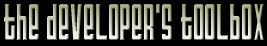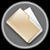This section of the archives stores flipcode's complete Developer Toolbox collection, featuring a variety of mini-articles and source code contributions from our readers.Faster Float-To-Int Conversion   Submitted byWarning: this COTD is seriously MSVC-only. It might apply to other compilers (in fact, I'm certain it does apply to, at least, other older compilers). But the implementation doesn't.

Warning: some assembly required if you want to understand how this is done.

Recently, two people have asked around me about the float-to-int conversion routines being very slow. This is a problem endemic to the way the Microsoft C library implements a little function called _ftol().

So, I've been trying out alternative implementations of this routine, and I've come up with several. I've been testing them on a 700 MHZ PC using this programs:

 ```/*Editor's note: COTD Entry: Faster Float-To-Int Conversion by JCAB [jcab@roningames.com] Warning: this COTD is seriously MSVC-only. It might apply to other compilers (in fact, I'm certain it does apply to, at least, other older compilers). But the implementation doesn't. Warning: some assembly required if you want to understand how this is done. Recently, two people have asked around me about the float-to-int conversion routines being very slow. This is a problem endemic to the way the Microsoft C library implements a little function called _ftol(). So, I've been trying out alternative implementations of this routine, and I've come up with several. I've been testing them on a 700 MHZ PC using this programs:*/// This first one checks the alternative implementation for correctness. // It needs the _ftol() function used (see below) rewritten as // "int FTOL(float)" and changed accordingly: volatile int k;int main(int argc, char* argv[]) { char s; enum { NUMTESTS = 10000000 }; static float rndbuf[NUMTESTS]; int i; for (i = 0; i < NUMTESTS; ++i) { int intg1 = rand(); int intg2 = rand(); int intg3 = rand(); int frac = rand(); rndbuf[i] = float(intg1) - float(intg2) + float(intg3) + float(frac) / RAND_MAX; } for (i = 0; i < NUMTESTS; ++i) { k = rndbuf[i]; if (k != FIST(rndbuf[i])) { sprintf(s, "Bad: %f (%08x) -> %d != %d\n", rndbuf[i], *(int*)&rndbuf[i], int(rndbuf[i]), FIST(rndbuf[i])); OutputDebugString(s); } } return 0; }// This program checks for performance: volatile int k;int main(int argc, char* argv[]) { char s; enum { NUMTESTS = 10000000 }; static float rndbuf[NUMTESTS]; int i; for (i = 0; i < NUMTESTS; ++i) { int intg1 = rand(); int intg2 = rand(); int intg3 = rand(); int frac = rand(); rndbuf[i] = float(intg1) - float(intg2) + float(intg3) + float(frac) / RAND_MAX; } DWORD time = GetTickCount(); int j; for (j = 0; j < 10; ++j) { for (i = 0; i < NUMTESTS; ++i) { k = rndbuf[i]; } } time = GetTickCount() - time; sprintf(s, "Time = %d ms\n", time); OutputDebugString(s); return 0; } The different functions I checked were:1- The compiler's own.2- Faster version that uses some global memory and requires rounding mode to be on: extern "C" __declspec(naked) void __cdecl _ftol() { const static int zpfp = { 0xBEFFFFFF, 0x3EFFFFFF }; __asm { SUB ESP,4 FST DWORD PTR [ESP] MOV EAX,DWORD PTR [ESP] SHR EAX,29 AND EAX,4 FADD DWORD PTR [zpfp+EAX] FISTP DWORD PTR [ESP] POP EAX RET } } 3- Slower version that uses no global memory and requires rounding mode to be on: extern "C" __declspec(naked) void __cdecl _ftol() { __asm { SUB ESP,4 FST DWORD PTR [ESP] MOV EAX,DWORD PTR [ESP] AND EAX,0x80000000 XOR EAX,0xBEFFFFFF MOV DWORD PTR [ESP],EAX FADD DWORD PTR [ESP] FISTP DWORD PTR [ESP] POP EAX RET } } 4- Version independent of the rounding mode, but which only works correctly on floats: extern "C" __declspec(naked) void __cdecl _ftol() { __asm { SUB ESP,4 FSTP DWORD PTR [ESP] POP EAX MOV EDX,EAX MOV ECX,EAX AND EAX,0x007FFFFF OR EAX,0x00800000 AND ECX,0x7F800000 JZ zero SHR ECX,23 SUB ECX,0x96 JC negexp SHL EAX,CL JMP shifted negexp: NEG ECX SHR EAX,CL shifted: AND EDX,0x80000000 JNZ negative RET negative: NEG EAX RET zero: SUB EAX,EAX RET } } 5- Version independent of the rounding mode, but which works correctly on doubles: extern "C" __declspec(naked) void __cdecl _ftol() { __asm { PUSH EDX PUSH ECX SUB ESP,8 FSTP QWORD PTR [ESP] POP EAX POP EDX MOV ECX,EDX AND EDX,0x000FFFFF OR EDX,0x00100000 SHL EDX,11 SHR EAX,21 OR EAX,EDX MOV EDX,ECX AND ECX,0x7FF00000 JZ zero SHR ECX,20 SUB ECX,0x41E JC negexp SHL EAX,CL JMP shifted negexp: NEG ECX CMP ECX,32 JAE zero SHR EAX,CL shifted: AND EDX,0x80000000 POP ECX POP EDX JNZ negative RET negative: NEG EAX RET zero: SUB EAX,EAX POP ECX POP EDX RET } } 6- Compiling with -QIfist, which doesn't return the correct result (it rounds, not chops). But it can be made to return the correct result if the rounding mode is changed at program startup and never modified afterwards. Note that I haven't made any efforts at optimizing the different routines. Specifically, might be possible to convert some jumps into CMOV instructions (PPro and up only). Note also that, in order for this replacement routines to work, they must be accessed before the one in the C library. The safest way to do it is to make sure it is in the same CPP file as the main() or WinMain() function. The timing results were: 1- Time = 10805 ms 2- Time = 3916 ms 3- Time = 4227 ms 4- Time = 4707 ms 5- Time = 7531 ms 6- Time = 2343 ms The different implementations have different trade-offs that make them all potentially viable for different developers. I'd like to see those functions optimized. If you do, please, send me a copy. ```